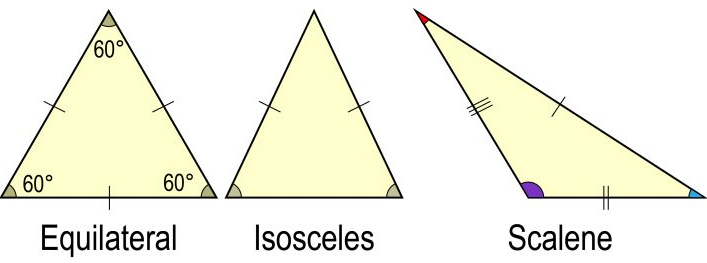# Properties of Triangle CalculatorGeometric Properties of a Triangle Side A: Area of Triangle: Side B: Area of Largest Inscribed Circle: Side C: Area of Smallest Inscribed Circle: Angle between A and B:

With this Calculator, you will not have to remember the complicated formulas for calculating the values of a triangle.This Calculator solves the simple values such as: area of triangle, area of largest/smallest inscribed circle, the angle between two side.

## What is Triangle?

a triangle is a plane figure, formed by three points called vertices, by the three segments which connect them, called sides, delimiting a domain of the plane called interior. When the vertices are distinct two by two, at each vertex the sides delimit an interior angle, from which the name “triangle” comes.

The triangle is also the simplest polygon that delimits a portion of the plane and thus serves as a fundamental element for the division and the approximation of surfaces.

## What are the properties of the triangle?

The sum of the angles of a triangle is 180°.

The angles of an equilateral triangle measure 60°.

The angles of the base of an isosceles triangle have the same measurement.

The sum of the acute angles of a right triangle is 90°

In any triangle ABC, we have the inequality: A B ≤ AC + BC..

## Calculate the area of a triangle

If c denotes the length of one side of a triangle and h the height relative to that side, the area of this triangle is equal to (c × h) ÷ 2.